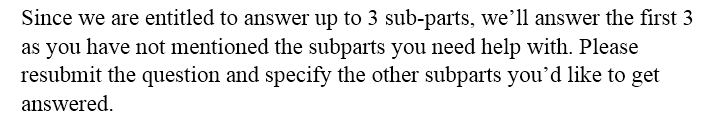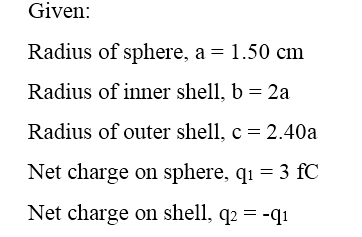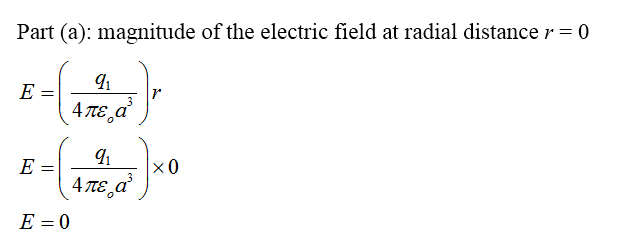# In the figure below, a solid sphere, of radius a = 1.50 cm is concentric with a spherical conducting shell of inner radius b = 2.00a and outer radius c = 2.40a. The sphere has a net charge q1 = +3.00 fC which is distributed uniformly through the sphere; the shell has a net charge of q2 = −q1.(a) What is the magnitude of the electric field at radial distance r = 0? _____________N/C(b) What is the magnitude of the electric field at radial distance r = a/4? ______________N/C(c) What is the magnitude of the electric field at radial distance r = a? ______________N/C(d) What is the magnitude of the electric field at radial distance r = 1.60a? _________________N/C(e) What is the magnitude of the electric field at radial distance r = 2.10a?________________ N/C(f) What is the magnitude of the electric field at radial distance r = 3.30a? _______________N/C(g) What is the net charge on the inner surface of the shell? _______________fC(h) What is the net charge on the outer surface of the shell? _______________fC

Question
18 views

In the figure below, a solid sphere, of radius a = 1.50 cm is concentric with a spherical conducting shell of inner radius b = 2.00a and outer radius c = 2.40a. The sphere has a net charge q1 = +3.00 fC which is distributed uniformly through the sphere; the shell has a net charge of q2 = −q1.

(a) What is the magnitude of the electric field at radial distance r = 0?
_____________N/C

(b) What is the magnitude of the electric field at radial distance r = a/4?
______________N/C

(c) What is the magnitude of the electric field at radial distance r = a?
______________N/C

(d) What is the magnitude of the electric field at radial distance r = 1.60a?
_________________N/C

(e) What is the magnitude of the electric field at radial distance r = 2.10a?
________________ N/C

(f) What is the magnitude of the electric field at radial distance r = 3.30a?
_______________N/C

(g) What is the net charge on the inner surface of the shell?
_______________fC

(h) What is the net charge on the outer surface of the shell?
_______________fC

check_circle

Step 1Step 2Step 3...

### Want to see the full answer?

See Solution

#### Want to see this answer and more?

Solutions are written by subject experts who are available 24/7. Questions are typically answered within 1 hour.*

See Solution
*Response times may vary by subject and question.
Tagged in